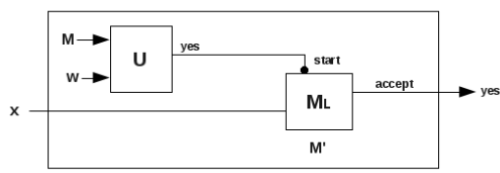Courses

# Rice Theorem Computer Science Engineering (CSE) Notes | EduRev

## Computer Science Engineering (CSE) : Rice Theorem Computer Science Engineering (CSE) Notes | EduRev

The document Rice Theorem Computer Science Engineering (CSE) Notes | EduRev is a part of the Computer Science Engineering (CSE) Course Theory of Computation.
All you need of Computer Science Engineering (CSE) at this link: Computer Science Engineering (CSE)

Part IX. Rice Theorem

Recursive and Non-Recursive Languages
Recursive Languages
A language, L is recursive, if
it is possible to design a TM that halts in the final state to say yes if w∈L, and halts in the non-final state to say no if w ∉ L.

Recursively Enumerable Languages
A language, L is recursively enumerable, if
it is possible to design a TM that halts in the final state to say yes if w∈L, and halting cannot be guranteed if w ∉ L.
Languages that are neither Recursive nor Recursively Enumerable
A language, L is neither recursive nor recursively enumerable, if the structure of the language is such that no TM which recognises w can be designed.

12 Rice Theorem
Rice’s theorem states that
every non-trivial property of a recursively enumerable language is undecidable.

Proof
A non-trivial property is one that is possessed by some objects of a class, but not all.
For example, being a mathematician is a property that is possessed by some humans but not by all.
Some cats are black but not all. So black colour property cannot be trivially associated with cats.
Let χ be a non-trivial property that is not possessed by all recursively enumerable languages. This problem can be reduced to one consisting of a pair (M, w) such that L possesses χ iff w ∈ L(M). We take a UTM U that takes a pair (M, w); its output is yes iff χ is possessed by L.
Since L is a recursively enumerable language, there must be a TM, ML that accepts L. Let x be a string belonging to L. Now, we design a machine M' to decide χ as shown below:Here U is a UTM.
UTM, U takes the pair (M, w) and checks if w ∈ L(M). If the output is yes, then the machine Mthat accepts the string x starts and the output of the machine M' is yes. Thus the decidability of the problem of possessing the trivial property reduces to the problem of Lu. If the pair (M, w) ∈ Lu, then L possesses χ ; otherwise not. Since Lu is not recursive,
possession of χ by L is also not decidable.

Offer running on EduRev: Apply code STAYHOME200 to get INR 200 off on our premium plan EduRev Infinity!

## Theory of Computation

18 videos|43 docs|39 tests

,

,

,

,

,

,

,

,

,

,

,

,

,

,

,

,

,

,

,

,

,

;